# Jointgrid

Inspired by the Seaborn jointgrid and @n_mondon charts, jointgrid gives a handy way to put marginal axis on each side of the pitch.

```import numpy as np
import pandas as pd
import seaborn as sns
from matplotlib.cm import get_cmap
import matplotlib.pyplot as plt

from mplsoccer import Pitch, VerticalPitch, Sbopen, FontManager

# get data for a Sevilla versus Barcelona match with a high amount of shots
parser = Sbopen()
df, related, freeze, tactics = parser.event(9860)

# setup the mplsoccer StatsBomb Pitches
# note not much padding around the pitch so the marginal axis are tight to the pitch
# if you are using a different goal type you will need to increase the padding to see the goals

fm = FontManager()
'RubikMonoOne-Regular.ttf')
```

Subset the shots for each team and move Barcelona’s shots to the other side of the pitch.

```# subset the shots
df_shots = df[df.type_name == 'Shot'].copy()

# subset the shots for each team
team1, team2 = df_shots.team_name.unique()
df_team1 = df_shots[df_shots.team_name == team1].copy()
df_team2 = df_shots[df_shots.team_name == team2].copy()

# Usually in football, the data is collected so the attacking direction is left to right.
# We can shift the coordinates via: new_x_coordinate = right_side - old_x_coordinate
# This is helpful for having one team shots on the left of the pitch and the other on the right
df_team1['x'] = pitch.dim.right - df_team1.x
```

## Plotting a standard shot map with step charts

```fig, axs = pitch.jointgrid(figheight=10,  # the figure is 10 inches high
left=None,  # joint grid center-aligned
bottom=0.075,  # grid starts 7.5% in from the bottom of the figure
marginal=0.1,  # marginal axes heights are 10% of grid height
space=0,  # 0% of the grid height reserved for space between axes
grid_width=0.9,  # the grid width takes up 90% of the figure width
title_height=0,  # plot without a title axes
axis=False,  # turn off title/ endnote/ marginal axes
endnote_height=0,  # plot without an endnote axes
grid_height=0.8)  # grid takes up 80% of the figure height
# we plot a usual scatter plot but the scatter size is based on expected goals
# note that the size is the expected goals * 700
# so any shots with an expected goals = 1 would take a size of 700 (points**2)
sc_team1 = pitch.scatter(df_team1.x, df_team1.y, s=df_team1.shot_statsbomb_xg * 700,
ec='black', color='#ba495c', ax=axs['pitch'])
sc_team2 = pitch.scatter(df_team2.x, df_team2.y, s=df_team2.shot_statsbomb_xg * 700,
ec='black', color='#697cd4', ax=axs['pitch'])
# (step) histograms on each of the left, top, and right marginal axes
team1_hist_y = sns.histplot(y=df_team1.y, ax=axs['left'], element='step', color='#ba495c')
team1_hist_x = sns.histplot(x=df_team1.x, ax=axs['top'], element='step', color='#ba495c')
team2_hist_x = sns.histplot(x=df_team2.x, ax=axs['top'], element='step', color='#697cd4')
team2_hist_y = sns.histplot(y=df_team2.y, ax=axs['right'], element='step', color='#697cd4')
txt1 = axs['pitch'].text(x=15, y=70, s=team1, fontproperties=fm.prop, color='#ba495c',
ha='center', va='center', fontsize=30)
txt2 = axs['pitch'].text(x=105, y=70, s=team2, fontproperties=fm.prop, color='#697cd4',
ha='center', va='center', fontsize=30)
```## Plotting a standard shot map with rug plots

```# decreased the marginal height as rug plots are only lines,
# we don't need as much space taken up by the marginal axes
fig, axs = pitch.jointgrid(figheight=10, left=None, bottom=0.075, marginal=0.02,
axis=False,  # turn off title/ endnote/ marginal axes
# plot without title/ endnote axes
endnote_height=0, title_height=0)
sc_team1 = pitch.scatter(df_team1.x, df_team1.y, s=df_team1.shot_statsbomb_xg * 700,
ec='black', color='#ba495c', ax=axs['pitch'])
sc_team2 = pitch.scatter(df_team2.x, df_team2.y, s=df_team2.shot_statsbomb_xg * 700,
ec='black', color='#697cd4', ax=axs['pitch'])
# note height=1 means that the whole of the marginal axes are taken up by the rugplots
team1_rug_y = sns.rugplot(y=df_team1.y, ax=axs['left'], color='#ba495c', height=1)
team1_rug_y = sns.rugplot(y=df_team2.y, ax=axs['right'], color='#697cd4', height=1)
team1_rug_x = sns.rugplot(x=df_team1.x, ax=axs['top'], color='#ba495c', height=1)
team2_rug_x = sns.rugplot(x=df_team2.x, ax=axs['top'], color='#697cd4', height=1)
txt1 = axs['pitch'].text(x=15, y=70, s=team1, fontproperties=fm.prop, color='#ba495c',
ha='center', va='center', fontsize=30)
txt2 = axs['pitch'].text(x=105, y=70, s=team2, fontproperties=fm.prop, color='#697cd4',
ha='center', va='center', fontsize=30)
```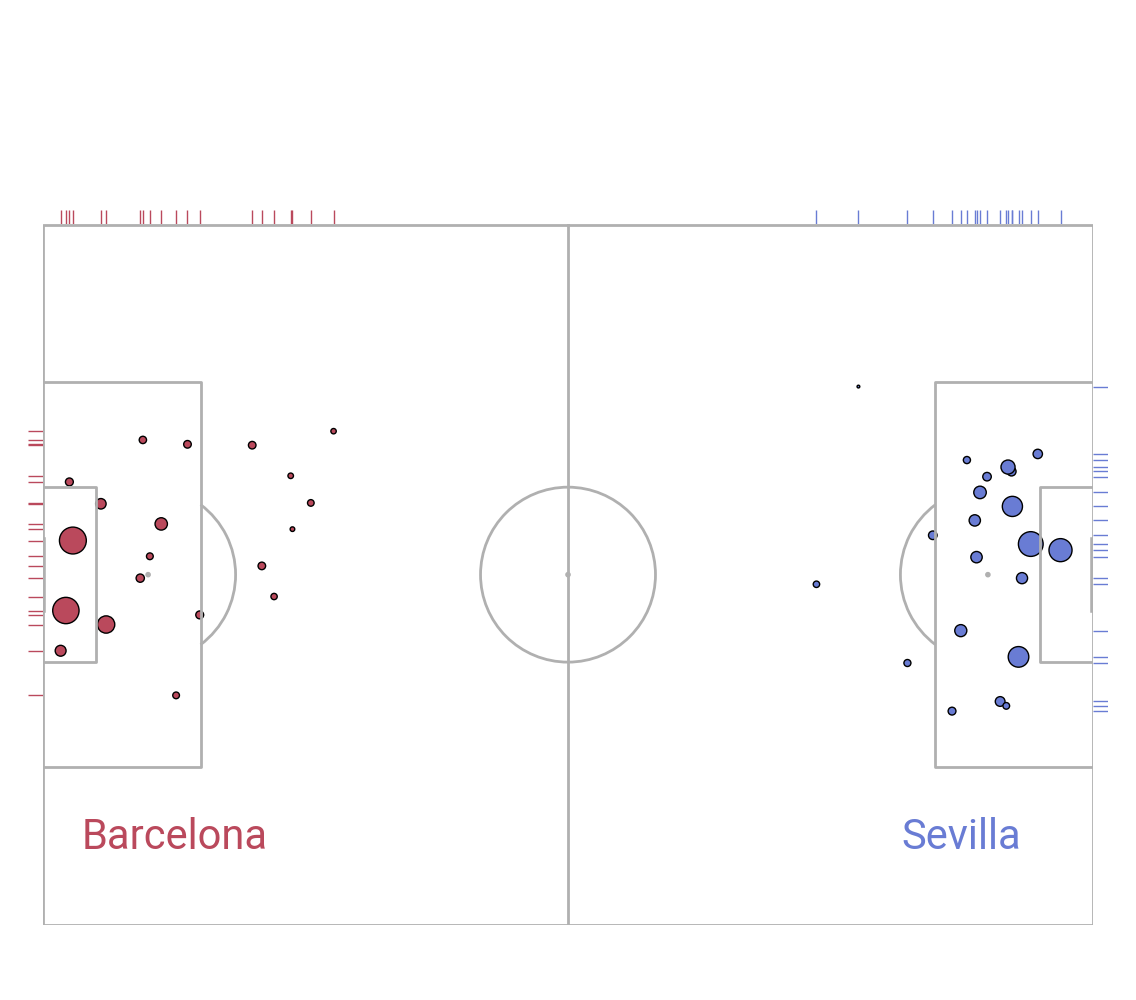Get more shot data for additional games

```# sevilla versus barcelona 2014/2015 to 2019/2020
match_files = [265835, 266142, 265839, 266989, 266280, 9673, 9860, 16029, 16190, 303473, 303674]
df = pd.concat([parser.event(file) for file in match_files])  # 0 index is the event file

# subset the shots
df_shots = df[df.type_name == 'Shot'].copy()

# subset the shots for each team
team1, team2 = df_shots.team_name.unique()
df_team1 = df_shots[df_shots.team_name == team1].copy().reset_index(drop=True)
df_team2 = df_shots[df_shots.team_name == team2].copy().reset_index(drop=True)

# move the team1 coordinate to the left hand side
df_team1['x'] = pitch.dim.right - df_team1.x
```

Get colors We are using Reds and Blues colormaps below and select a color just over half way (60%) through the colormap for use in the charts.

```red = get_cmap('Reds')(np.linspace(0, 1, 100))
blue = get_cmap('Blues')(np.linspace(0, 1, 100))
```
```/home/docs/checkouts/readthedocs.org/user_builds/mplsoccer/checkouts/latest/examples/pitch_plots/plot_jointgrid.py:128: MatplotlibDeprecationWarning: The get_cmap function was deprecated in Matplotlib 3.7 and will be removed two minor releases later. Use ``matplotlib.colormaps[name]`` or ``matplotlib.colormaps.get_cmap(obj)`` instead.
red = get_cmap('Reds')(np.linspace(0, 1, 100))
/home/docs/checkouts/readthedocs.org/user_builds/mplsoccer/checkouts/latest/examples/pitch_plots/plot_jointgrid.py:129: MatplotlibDeprecationWarning: The get_cmap function was deprecated in Matplotlib 3.7 and will be removed two minor releases later. Use ``matplotlib.colormaps[name]`` or ``matplotlib.colormaps.get_cmap(obj)`` instead.
blue = get_cmap('Blues')(np.linspace(0, 1, 100))
```

## Hexbin shot map with kdeplot marginal axes

```fig, axs = pitch.jointgrid(figheight=10, left=None, bottom=0.075, grid_height=0.8,
axis=False,  # turn off title/ endnote/ marginal axes
# plot without endnote/ title axes
endnote_height=0, title_height=0)
# plot the hexbins
hex1 = pitch.hexbin(df_team1.x, df_team1.y, ax=axs['pitch'],
edgecolors=pitch.line_color, cmap='Reds')
hex2 = pitch.hexbin(df_team2.x, df_team2.y, ax=axs['pitch'],
edgecolors=pitch.line_color, cmap='Blues')
# normalize the values so the colors depend on the minimum/ value for both teams
# this ensures that darker colors mean more shots relative to both teams
vmin = min(hex1.get_array().min(), hex2.get_array().min())
vmax = max(hex1.get_array().max(), hex2.get_array().max())
hex1.set_clim(vmin=vmin, vmax=vmax)
hex2.set_clim(vmin=vmin, vmax=vmax)
# plot kdeplots on the marginals
team1_hist_y = sns.kdeplot(y=df_team1.y, ax=axs['left'], color=red, fill=True)
team1_hist_x = sns.kdeplot(x=df_team1.x, ax=axs['top'], color=red, fill=True)
team2_hist_x = sns.kdeplot(x=df_team2.x, ax=axs['top'], color=blue, fill=True)
team2_hist_y = sns.kdeplot(y=df_team2.y, ax=axs['right'], color=blue, fill=True)
txt1 = axs['pitch'].text(x=15, y=70, s=team1, fontproperties=fm.prop, color=red,
ha='center', va='center', fontsize=30)
txt2 = axs['pitch'].text(x=105, y=70, s=team2, fontproperties=fm.prop, color=blue,
ha='center', va='center', fontsize=30)
```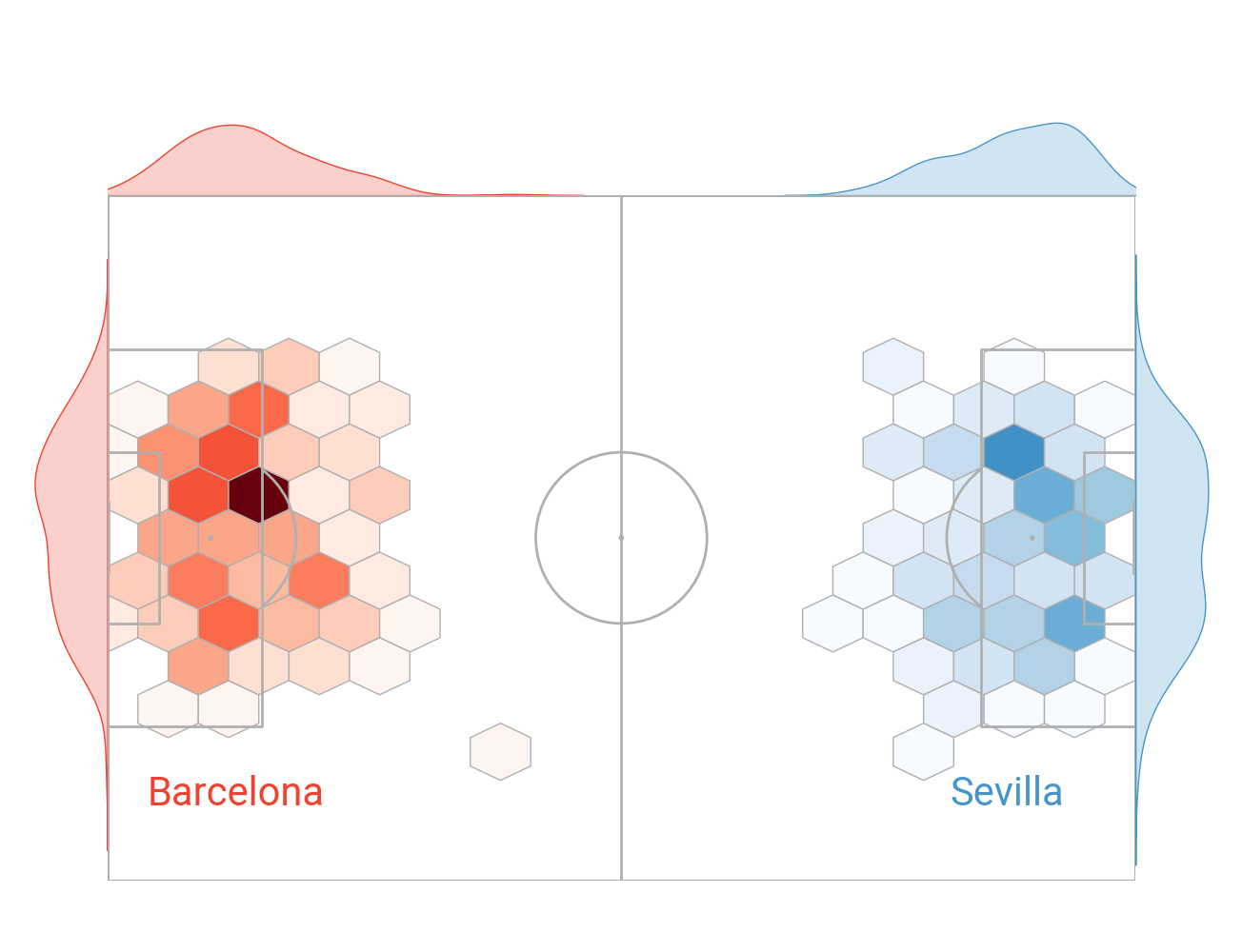## Heatmap shot map with histogram/ kdeplot on the marginal axes

```fig, axs = pitch.jointgrid(figheight=10, left=None, bottom=0.075, grid_height=0.8,
axis=False,  # turn off title/ endnote/ marginal axes
# plot without endnote/ title axes
title_height=0, endnote_height=0)
bs1 = pitch.bin_statistic(df_team1.x, df_team1.y, bins=(18, 12))
bs2 = pitch.bin_statistic(df_team2.x, df_team2.y, bins=(18, 12))
# get the min/ max values for normalizing across both teams
vmax = max(bs2['statistic'].max(), bs1['statistic'].max())
vmin = max(bs2['statistic'].min(), bs1['statistic'].min())
# set values where zero shots to nan values so it does not show up in the heatmap
# i.e. zero values take the background color
bs1['statistic'][bs1['statistic'] == 0] = np.nan
bs2['statistic'][bs2['statistic'] == 0] = np.nan
# set the vmin/ vmax so the colors depend on the minimum/maximum value for both teams
hm1 = pitch.heatmap(bs1, ax=axs['pitch'], cmap='Reds', vmin=vmin, vmax=vmax, edgecolor='#f9f9f9')
hm2 = pitch.heatmap(bs2, ax=axs['pitch'], cmap='Blues', vmin=vmin, vmax=vmax, edgecolor='#f9f9f9')
# histograms with kdeplot
team1_hist_y = sns.histplot(y=df_team1.y, ax=axs['left'], color=red, linewidth=1, kde=True)
team1_hist_x = sns.histplot(x=df_team1.x, ax=axs['top'], color=red, linewidth=1, kde=True)
team2_hist_x = sns.histplot(x=df_team2.x, ax=axs['top'], color=blue, linewidth=1, kde=True)
team2_hist_y = sns.histplot(y=df_team2.y, ax=axs['right'], color=blue, linewidth=1, kde=True)
txt1 = axs['pitch'].text(x=15, y=70, s=team1, fontproperties=fm.prop, color=red,
ha='center', va='center', fontsize=30)
txt2 = axs['pitch'].text(x=105, y=70, s=team2, fontproperties=fm.prop, color=blue,
ha='center', va='center', fontsize=30)
```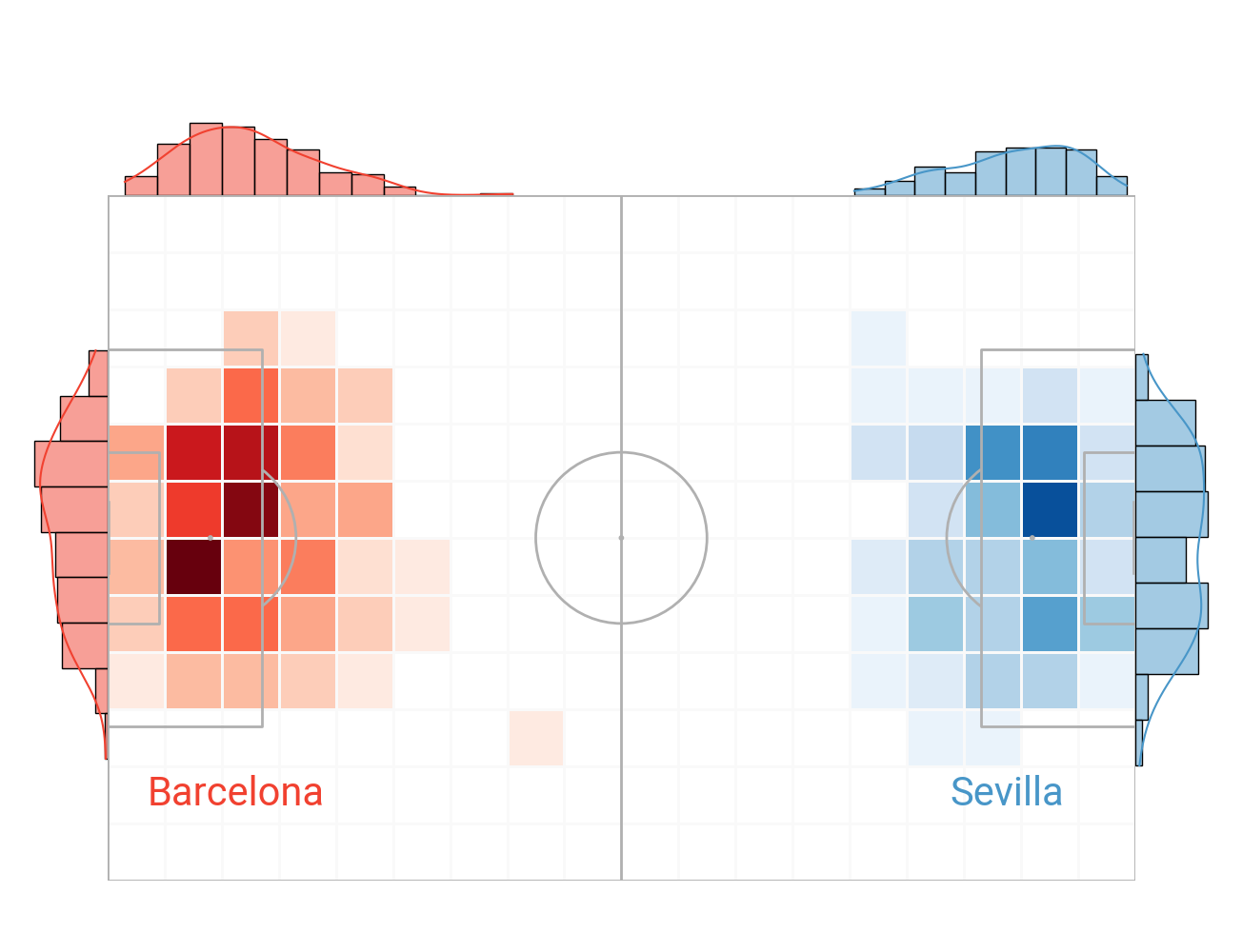## Kdeplot shot map with kdeplot on the marginal axes

```fig, axs = pitch.jointgrid(figheight=10, left=None, bottom=0.075, grid_height=0.8,
axis=False,  # turn off title/ endnote/ marginal axes
# plot without endnote/ title axes
title_height=0, endnote_height=0)
# increase number of levels for a smoother looking heatmap
kde1 = pitch.kdeplot(df_team1.x, df_team1.y, ax=axs['pitch'], cmap='Reds', levels=75, fill=True)
kde2 = pitch.kdeplot(df_team2.x, df_team2.y, ax=axs['pitch'], cmap='Blues', levels=75, fill=True)
# kdeplot on marginal axes
team1_hist_y = sns.kdeplot(y=df_team1.y, ax=axs['left'], color=red, fill=True)
team1_hist_x = sns.kdeplot(x=df_team1.x, ax=axs['top'], color=red, fill=True)
team2_hist_x = sns.kdeplot(x=df_team2.x, ax=axs['top'], color=blue, fill=True)
team2_hist_y = sns.kdeplot(y=df_team2.y, ax=axs['right'], color=blue, fill=True)
txt1 = axs['pitch'].text(x=15, y=70, s=team1, fontproperties=fm.prop, color=red,
ha='center', va='center', fontsize=30)
txt2 = axs['pitch'].text(x=105, y=70, s=team2, fontproperties=fm.prop, color=blue,
ha='center', va='center', fontsize=30)
```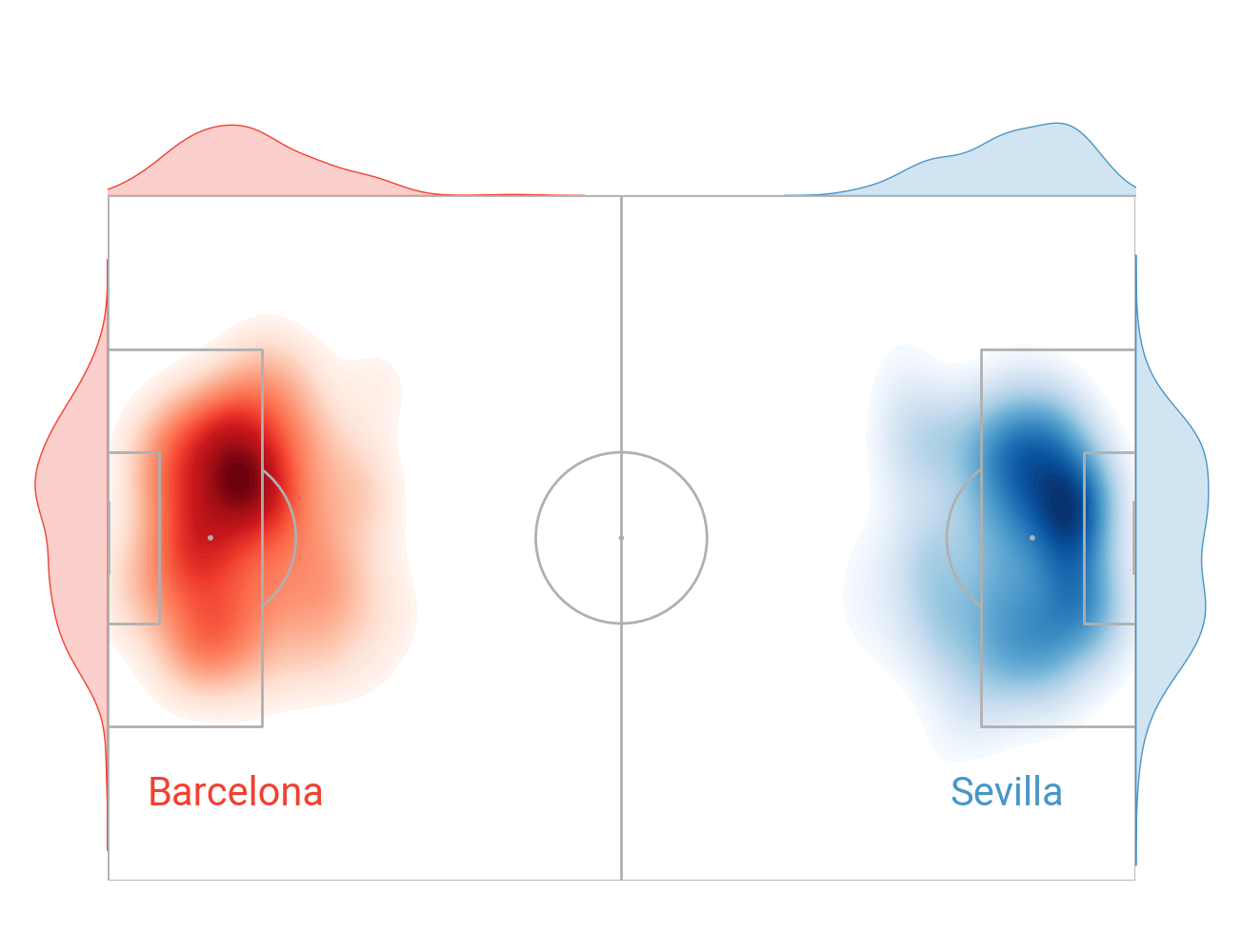## Vertical shot map with kdeplot marginals

The jointgrid is flexible. You can filter the marginal axes with ax_left, ax_top, ax_left, ax_right. Here we set the bottom and right marginal axes to display for a single team.

```fig, axs = vertical_pitch.jointgrid(figheight=10, left=None, bottom=0.15,
grid_height=0.7, marginal=0.1,
# plot without endnote/ title axes
endnote_height=0, title_height=0,
axis=False,  # turn off title/ endnote/ marginal axes
# here we filter out the left and top marginal axes
ax_top=False, ax_bottom=True,
ax_left=False, ax_right=True)
# typical shot map where the scatter points vary by the expected goals value
# using alpha for transparency as there are a lot of shots stacked around the six-yard box
sc_team2 = vertical_pitch.scatter(df_team2.x, df_team2.y, s=df_team2.shot_statsbomb_xg * 700,
alpha=0.5, ec='black', color='#697cd4', ax=axs['pitch'])
# kdeplots on the marginals
# remember to flip the coordinates y=x, x=y for the marginals when using vertical orientation
team2_hist_x = sns.kdeplot(y=df_team2.x, ax=axs['right'], color='#697cd4', fill=True)
team2_hist_y = sns.kdeplot(x=df_team2.y, ax=axs['bottom'], color='#697cd4', fill=True)
txt1 = axs['pitch'].text(x=40, y=80, s=team2, fontproperties=fm_rubik.prop, color=pitch.line_color,
ha='center', va='center', fontsize=60)
```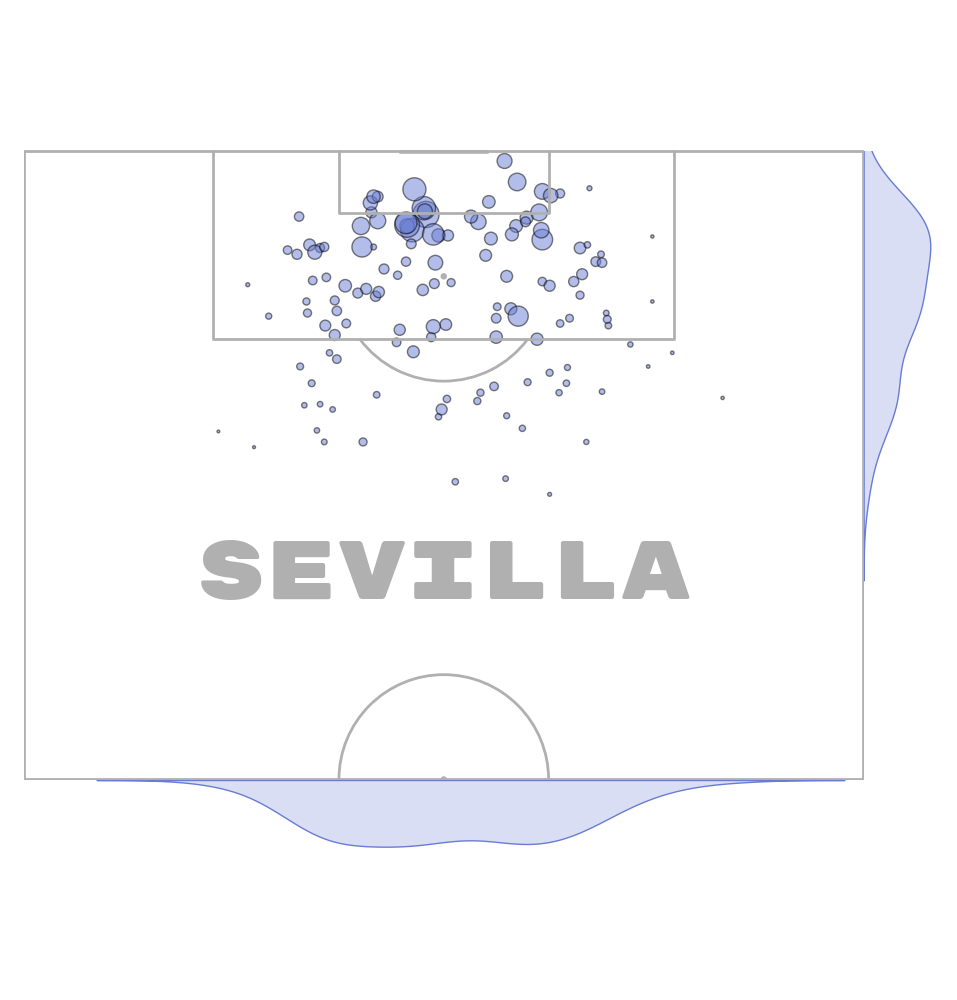## Crop the pitch

The jointgrid also works with arbritary padding. So you can crop the pitc and still have the marginal axes to plot on.

```vertical_pitch = VerticalPitch(half=True,
# here we remove some of the pitch on the left/ right/ bottom
goal_type='line')

fig, axs = vertical_pitch.jointgrid(figheight=10, left=None, bottom=0.15,
grid_height=0.7, marginal=0.1,
# plot without an endnote/ title axes
title_height=0, endnote_height=0,
axis=False,  # turn off title/ endnote/ marginal axes
# here we filter out the left and top marginal axes
ax_top=False, ax_bottom=True,
ax_left=False, ax_right=True)
# typical shot map where the scatter points vary by the expected goals value
# using alpha for transparency as there are a lot of shots stacked around the six-yard box
sc_team2 = vertical_pitch.scatter(df_team2.x, df_team2.y, s=df_team2.shot_statsbomb_xg * 700,
alpha=0.5, ec='black', color='#697cd4', ax=axs['pitch'])
# kdeplots on the marginals
# remember to flip the coordinates y=x, x=y for the marginals when using vertical orientation
team2_hist_x = sns.kdeplot(y=df_team2.x, ax=axs['right'], color='#697cd4', fill=True)
team2_hist_y = sns.kdeplot(x=df_team2.y, ax=axs['bottom'], color='#697cd4', fill=True)
txt1 = axs['pitch'].text(x=40, y=85, s=team2, fontproperties=fm_rubik.prop, color=pitch.line_color,
ha='center', va='center', fontsize=60)
```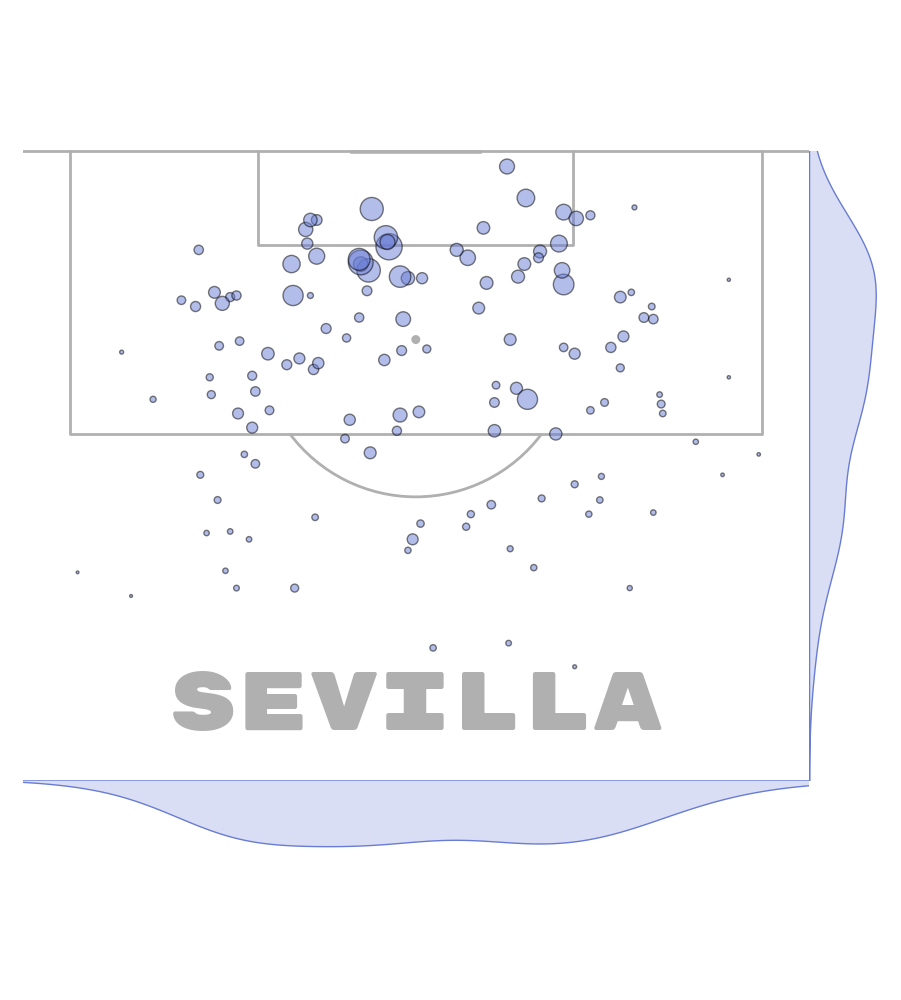## Add a title and endnote

The jointgrid also has an option to plot an endnote and a title axes.

```vertical_pitch = VerticalPitch(half=True,
# here we remove some of the pitch on the left/ right/ bottom
goal_type='line')

fig, axs = vertical_pitch.jointgrid(figheight=10, left=None, bottom=None,  # center aligned
grid_width=0.95, marginal=0.1,
# setting up the heights/space so it takes up 95% of the figure
grid_height=0.80,
title_height=0.1, endnote_height=0.03,
title_space=0.01, endnote_space=0.01,
axis=False,  # turn off title/ endnote/ marginal axes
# here we filter out the left and top marginal axes
ax_top=False, ax_bottom=True,
ax_left=False, ax_right=True)
# typical shot map where the scatter points vary by the expected goals value
# using alpha for transparency as there are a lot of shots stacked around the six-yard box
sc_team2 = vertical_pitch.scatter(df_team2.x, df_team2.y, s=df_team2.shot_statsbomb_xg * 700,
alpha=0.5, ec='black', color='#697cd4', ax=axs['pitch'])
# kdeplots on the marginals
# remember to flip the coordinates y=x, x=y for the marginals when using vertical orientation
team2_hist_x = sns.kdeplot(y=df_team2.x, ax=axs['right'], color='#697cd4', fill=True)
team2_hist_y = sns.kdeplot(x=df_team2.y, ax=axs['bottom'], color='#697cd4', fill=True)
txt1 = axs['pitch'].text(x=40, y=85, s=team2, fontproperties=fm_rubik.prop, color=pitch.line_color,
ha='center', va='center', fontsize=60)

# titles and endnote
axs['title'].text(0.5, 0.7, "Sevilla's shots versus Barcelona", color=pitch.line_color,
fontproperties=fm_rubik.prop, fontsize=18, ha='center', va='center')
axs['title'].text(0.5, 0.3, "2014/15 to 2019/20", color=pitch.line_color,
fontproperties=fm_rubik.prop, fontsize=12, ha='center', va='center')
axs['endnote'].text(1, 0.5, '@your_amazing_tag', ha='right', va='center',
color=pitch.line_color, fontproperties=fm_rubik.prop)

plt.show()  # If you are using a Jupyter notebook you do not need this line
```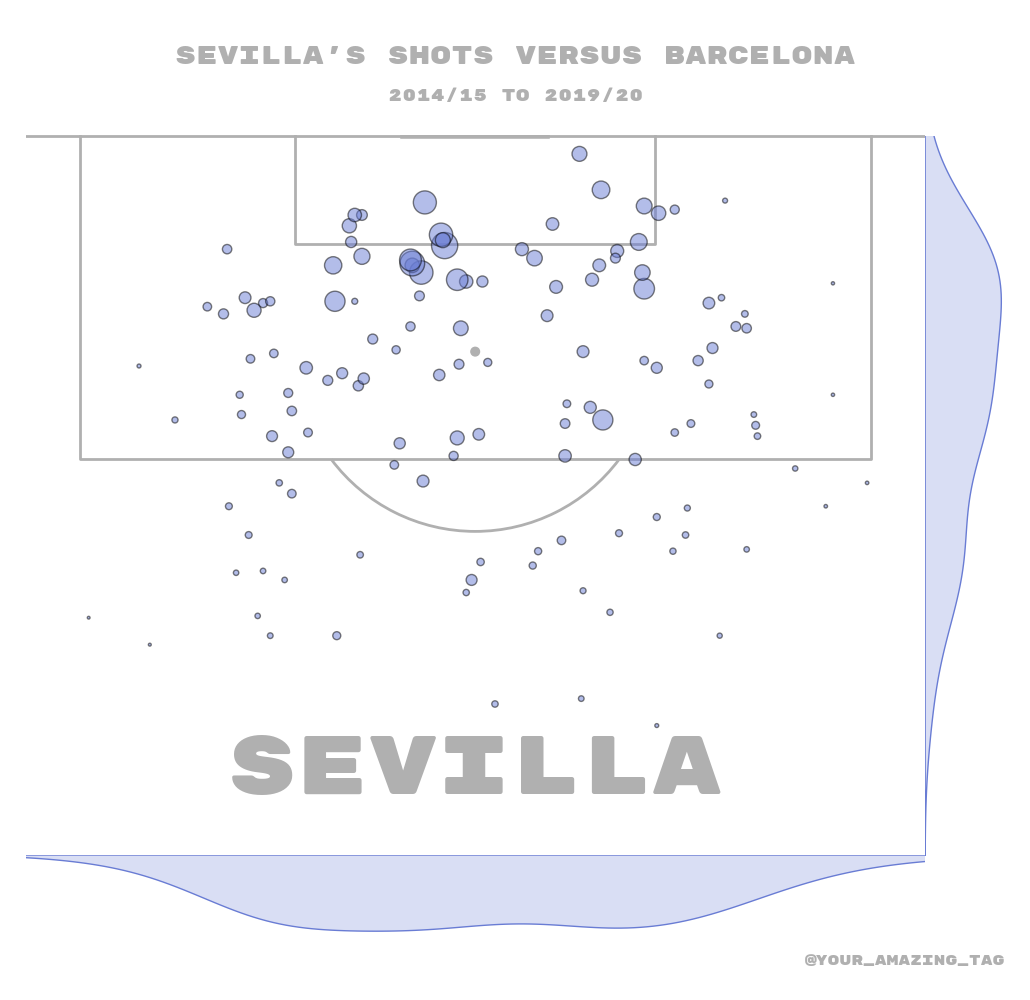Total running time of the script: ( 0 minutes 10.903 seconds)

Gallery generated by Sphinx-Gallery